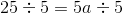# ISEE Middle Level Math : Equations

## Example Questions

← Previous 1 3 4 5 6 7 8 9 41 42

### Example Question #1 : Equations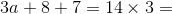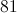Explanation:

To solve:

First, solve both equations on each end, keeping the variable.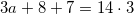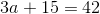Then, subtract 15 from each side of the equation.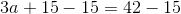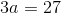Finally, divide each side by 3 so that only the variable remains.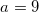### Example Question #3 : How To Solve One Step Equations

Ann, Bailey, Cara, Jack, and Jill are running a marathon.  Cara finishes 4 minutes before Ann.  Jill finishes after Bailey, who finishes after Ann.  Jack finishes right before Bailey.  Who else does Jack finish the race before?

Ann

Jill

Cara

There is not enough information to solve this problem.

Bailey

Jill

Explanation:

Cara finishes first.  Ann and Bailey finish before Jill.  If Jack finishes right before Bailey, then Jill finishes last.

### Example Question #1 : Equations

Solve for: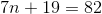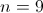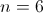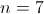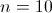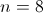Explanation:

Subtract 19, then divide by 7: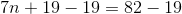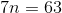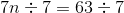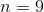### Example Question #1 : How To Find The Solution To An Equation

A bicycle originally costs $120.00. If it is on sale for 30% off, what is the sale price of the bicycle? Possible Answers: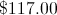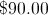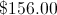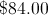Correct answer:Explanation: First multiply the original price by 30%.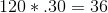Then subtract that amount from the original price.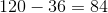The sale price is$84.00

### Example Question #211 : Algebraic Concepts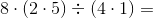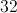Explanation:

First solve the parentheses.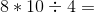Then solve from left to right since multiplication and division are interchangeable: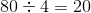### Example Question #212 : Algebraic Concepts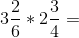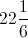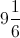Explanation:

First, convert each mixed number into an improper fraction.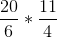Then, reduce where possible and multiply.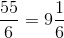### Example Question #1 : How To Find The Solution To An Equation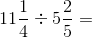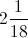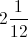Explanation:

First, convert each mixed number into an improper fraction and flip the second fraction. Then, change the operation to multiplication: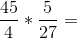Reduce where possible and multiply. Convert your answer into a mixed fraction.

### Example Question #2 : How To Find The Solution To An Equation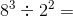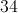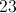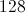Explanation:

First find the exponent value for each number: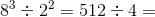Then divide accordingly. The answer is 128.

### Example Question #821 : Concepts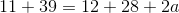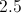Explanation:

First follow order of operations and solve on each side.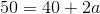Then, subtract 40 from each side to leave only the variable: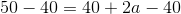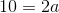Finally, divide both sides by 2.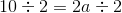The answer is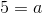### Example Question #2 : How To Find The Solution To An Equation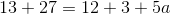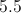Explanation:

First solve with order of operations on each side: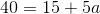Then subtract 15 from each side: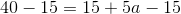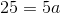Finally, divide each side by 5: